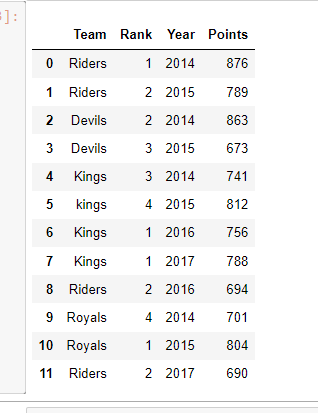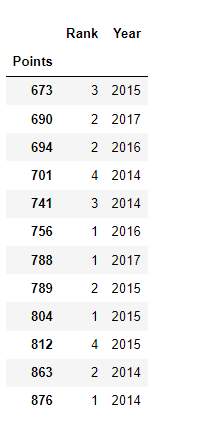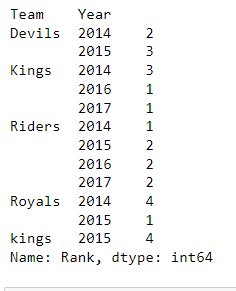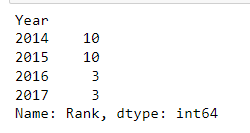Open In App

# Pandas Groupby and Sum

It’s a simple concept but it’s an extremely valuable technique that’s widely used in data science. It is helpful in the sense that we can :

• Compute summary statistics for every group
• Perform group-specific transformations
• Do the filtration of data

The dataframe.groupby() involves a combination of splitting the object, applying a function, and combining the results. This can be used to group large amounts of data and compute operations on these groups such as sum().

Pandas dataframe.sum() function returns the sum of the values for the requested axis. If the input is the index axis then it adds all the values in a column and repeats the same for all the columns and returns a series containing the sum of all the values in each column.

## Python3

 `# import required module``import` `pandas as pd` `# assign data``ipl_data ``=` `{``'Team'``: [``'Riders'``, ``'Riders'``, ``'Devils'``, ``'Devils'``,``                     ``'Kings'``,  ``'kings'``, ``'Kings'``, ``'Kings'``,``                     ``'Riders'``, ``'Royals'``, ``'Royals'``, ``'Riders'``],``            ``'Rank'``: [``1``, ``2``, ``2``, ``3``, ``3``, ``4``, ``1``, ``1``, ``2``, ``4``, ``1``, ``2``],` `            ``'Year'``: [``2014``, ``2015``, ``2014``, ``2015``, ``2014``, ``2015``, ``2016``,``                     ``2017``, ``2016``, ``2014``, ``2015``, ``2017``],` `            ``'Points'``: [``876``, ``789``, ``863``, ``673``, ``741``, ``812``, ``756``, ``788``,``                       ``694``, ``701``, ``804``, ``690``]}` `# create dataframe``df ``=` `pd.DataFrame(ipl_data)`

Output:Example 1: Pandas groupby() & sum() by Column Name

In this example, we group data on the Points column and calculate the sum for all numeric columns of DataFrame.

## Python3

 `# use groupby() to compute sum``df.groupby([``'Points'``]).``sum``()`

Output:Example 2: Pandas groupby() & sum() on Multiple Columns

Here, we can apply a group on multiple columns and calculate a sum over each combination group.

## Python3

 `# use groupby() to generate sum``df.groupby([``'Team'``, ``'Year'``])[``'Rank'``].``sum``()`

Output:Example 3: Sort order by groupby Keys

In this example, we group data on the Year column and calculate the sum for all numeric columns of DataFrame, and also sort Year in ascending order.

## Python3

 `# use groupby() to generate sum``df.groupby([``'Year'``], sort``=``True``)[``'Rank'``].``sum``()`

Output: